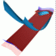Abstract and Contents
Up: 3. The algorithm
Next: 3.3 The case of a hyperbolic fixed point
Previous: 3.1 Iterating a fundamental domain

# 3.2 Globalization by adding discrete circles

The algorithm produces a mesh of points on the unstable manifold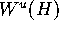. This mesh is a discrete object, and it is represented by sequences of N+1 points, where each sequence is a discretization of a piece of the curvein each of the leaves of. We desribe the algorithm in its simplest form, when the number of leaves |M| remains fixed. Mesh adaptation by adding leaves during the computation is the topic of Section 3.4.

The mesh is computed in steps by adding a (discrete) circle to the mesh of the previous step. This process is illustrated with an animation. By a (discrete) circle we mean a set of |M| points, one in each leaf of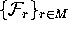. The algorithm stops when the prescribed number N of circles has been added. The mesh is stored in the array `Mesh[i][`r`]` of points in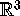, where `i`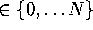and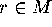. In other words, `Mesh[i]` is a circle of |M| points, and `Mesh[*][`r`]` is a seqence of N+1 points in the leaf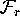. The initial circle `Mesh` is simply M, and the next circle `Mesh` consists of the points that are a prescribed initial distancefrom M along the linear approximation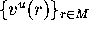.

During the computation we need the continuous object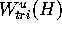, which is the approximation of the computed part ofdefined as a linear triangulation of the mesh points.is stored as the dynamical array of triangles `Tri`. Each triangle contains three pointers to points of `Mesh` and three pointers to its direct neighbors. Note that consecutive circles of points in `Mesh` define a ring of triangles in `Tri` of. Consequently, adding a circle to `Mesh` means adding a ring to `Tri`.

Suppose that we want to add a circle to `Mesh`, which means that we want to add a new point to each of the |M| sequences `Mesh[*][`r`]`. Such a point is the next point along the curveat a prescribed distance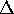from the last point. The basic idea is to find a point q in the continuous objectthat maps intounder f and lies at distancefrom the last point in. Sinceis close to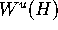, its imageis close toas well. In practical terms, we need to find the triangle T in `Tri` that contains q, and then find q by bisection in T. This works if the distance between the last circle and its f-image is at least; see Figure 2 (bottom).

We now give a detailed, more technical description of the algorithm. It can be found in pseudo-code in Figure 4 and Figure 5. Suppose that we have computed k-1 rings that are stored as a mesh of k circles in `Mesh[i][`r`]`, where `i`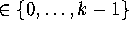, and let `Tri` contain all triangles of the associated triangulation. The idea is to add a new circle `Mesh[k]` of points at a prescribed distancefrom the last circle `Mesh[k-1]`. This can be done if the distance between `Mesh[k-1]` and its f-image is at least; compare Figure 2 (bottom). If this distance is less thanwe add a new circle at this smaller distance from `Mesh[k-1]`. The latter situation is certain to occur in the beginning of a computation, when the distance of `Mesh[k-1]` to H is still small.

Adding the circle `Mesh[k]` to the mesh means that for all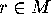we need to find one additional point `Mesh[k][`r`]` in each sequence `Mesh[*][`r`]`. This new point must lie at distancefrom `Mesh[k-1][`r`]` along the unique intersection curve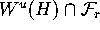. The idea is to find a point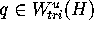, such that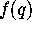is this new point in the sequence; see Figure 6. Clearly q must satisfy two conditions: it must map into, and it must map to a point of distancefrom `Mesh[k-1][`r`]`. The new point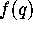lies close to, because.

In most cases, the intersectionis a single connected curve, so that T contains the point q we are looking for. This situation is sketched in Figure 7 (top). By (two-dimensional) bisection on T the point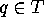can be found on the curve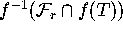. The bisection is exact up to the prescribed bisection tolerance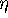. We then set `Mesh[k][`r`]` =. It may happen thatconsists of two separate curves, so that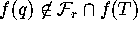and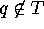. Then typically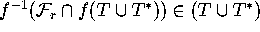. Here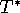is the (uniquely defined) triangle, which has the edge with T in common that is not entered first or exited last by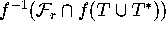; see Figure 7 (bottom). In this case, q can be found by bisection on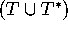. In our implementation, we found it sucessful to always perfom bisection on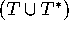fo find q. In case a point q with the mentioned properties cannot be found, the algorithm stops. This may be caused by the fact that also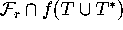consists of disconnected pieces. If this is indeed the case it is possible to continue the computation further by restarting with smaller.

The algorithm GLOBALIZE is shown in pseudo-code in Figure 4. The procedure MINDIST computes the minimal distance between f(`Mesh[k-1]`) and `Mesh[k-1]`. This is done by first computing the intersections ofwith the f-image of the continuous polygon with vertices `Mesh[k-1]`, and then taking the minimum of the respective distances in the leaves. The heart of the algorithm is the procedure ADDCIRCLE, which returns the new circle `Mesh[k]`. Then `Tri` is updated by UPDATE, which means that a new ring of triangles is added to `Tri`. ADDCIRCLE is shown in pseudo-code in Figure 5. The procedure FINDTRIANGLE returns the triangle T as specified above, and the procedure BISECTION returns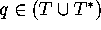. This completes the description of the algorithm.

Up: 3. The algorithm
Next: 3.3 The case of a hyperbolic fixed point
Previous: 3.1 Iterating a fundamental domainAbstract and Contents

Written by: Bernd Krauskopf & Hinke Osinga
Created: May 27 1997 --- Last modified: Wed Jul 2 10:51:06 1997